Wir verwenden Google für unsere Suche. Mit Klick auf „Suche aktivieren“ aktivieren Sie das Suchfeld und akzeptieren die Nutzungsbedingungen.

# New in SG-Lib 3.8

###### VLdistance(VL)- returns a distance list - but is different from VLnorm% VLdistance(VL) - returns a distance list - but is different from VLnorm% (by Tim Lueth, VLFL-Lib, 2017-MAI-24 as class: ANALYTICAL GEOMETRY)% % Version 4.0 is 10-20 times faster than 3.9% VLnorm - vertex normal vector; not defined for the first value% VLdistance - vertex distance vector; not defined for the last value% The results seem to be circulated by one% VLdistance is looking forward% VLnorm is looking backward (Status of: 2017-07-09)% % Introduced first in SolidGeometry 3.8% % See also: NLnorm, VLangle% % [DL,DVL]=VLdistance(VL)% === INPUT PARAMETERS ===% VL: Vertex list% === OUTPUT RESULTS ======% DL: Distance list% DVL: Distance vector list% % EXAMPLE: % VLnorm(VLsample(3))% VLdistance(VLsample(3))% % See also: NLnorm, VLangle%
###### PLof3dist(d1,d2,d3,srt)- creates a 3P Tracker geometry using the given distances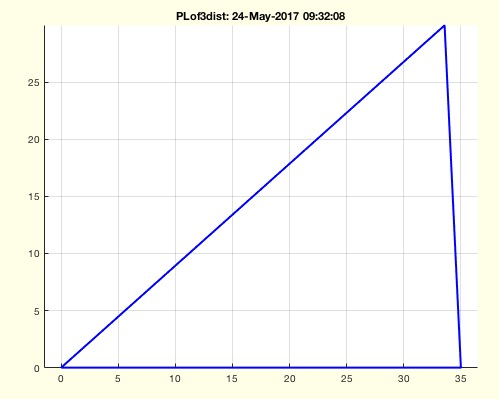% PLof3dist(d1,d2,d3,srt) - creates a 3P Tracker geometry using the given distances% (by Tim Lueth, VLFL-Lib, 2017-MAI-24 as class: ANALYTICAL GEOMETRY)% % See also: cross2circ% % [PL,n,d3]=PLof3dist(d1,d2,[d3,srt])% === INPUT PARAMETERS ===% d1: distance in x% d2: radius at second point% d3: radius at third/first point point;% srt: sort by length; default is false% === OUTPUT RESULTS ======% PL: Point list% n: area of triangle% d3: d3% % EXAMPLE: % PLof3dist(35,30,45)% PLof3dist(35,30,45,true)%% SGspherelink(Lz,R1,R2,olap) - returns a spherical link% (by Tim Lueth, VLFL-Lib, 2017-MAI-18 as class: SURFACES)% % This fnctn is helpful to design more natural shaped links (Status of: % 2017-05-18)% % See also: SGsphere% % SGC=SGspherelink(Lz,[R1,R2,olap])% === INPUT PARAMETERS ===% Lz: Length in Z% R1: Upper radial values [Rx Ry Rz]; default is L/4% R2: lower radial values [Rx Ry Rz]; default is R1% olap: overlap of the three solids; default is 1e-3;% === OUTPUT RESULTS ======% SGC: Final solid% % EXAMPLE: % SGspherelink(20,[5 5 5]); % Pill shape% SGspherelink(20,[5 5 0]); % Cylinder shape% SGspherelink(20,[5 2 0]); % Rod% SGspherelink(20,[5 5 10],[5 5 0]); % Jules Verne Moon Rocket Shape% SGspherelink(450,[400 200 80],[300 150 0]); % Torso shape%
###### CVLofSGslices(SG,n)- returns slices as CVL (CPL including z) of a SG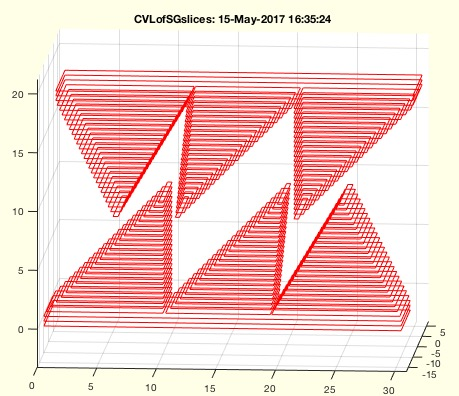% CVLofSGslices(SG,n) - returns slices as CVL (CPL including z) of a SG % (by Tim Lueth, VLFL-Lib, 2017-MAI-15 as class: SLICES)% % This fnctn is useful for creating 3D printing strategies over several % slices. It also helps to reconstruct solids (Status of: 2017-05-26)% % Introduced first in SolidGeometry 3.8% % See also: CPLofSGslice3, SGofCVLslices, CVLseparate% % CVL=CVLofSGslices(SG,[n])% === INPUT PARAMETERS ===% SG: Solid Geometry% n: number of slices; default is 10% === OUTPUT RESULTS ======% CVL: CPL of the slices including the relevant c coordinate% % EXAMPLE: % CVLofSGslices(SGsample(23),50)% % See also: CPLofSGslice3, SGofCVLslices, CVLseparate%
###### SGunitecells(SGc)- converts all cells into one solid geometry% SGunitecells(SGc) - converts all cells into one solid geometry% (by Tim Lueth, VLFL-Lib, 2017-MAI-15 as class: SURFACES)% % Quick and Dirty - Does not support frames yet% (Status of: 2017-05-19)% % See also: SGcat, SGcat2, VLFLofSG, SGofVLFL% % SG=SGunitecells(SGc)% === INPUT PARAMETERS ===% SGc: {SGA; SGB; SGC;}% === OUTPUT RESULTS ======% SG: SG.VL, SG.FL% % EXAMPLE: % SGunitecells(SGsample(22))% SGunitecells(SGsample(23))%
###### PLofimcontourc(I,cent,pixs)- returns the centers of pixel coordinates in an image% PLofimcontourc(I,cent,pixs) - returns the centers of pixel coordinates in an image% (by Tim Lueth, VLFL-Lib, 2017-MAI-14 as class: CLOSED POLYGON LISTS)% % See also: CPLcontourc% % PL=PLofimcontourc(I,[cent,pixs])% === INPUT PARAMETERS ===% I: Image% cent: true= image origin is in center; default is false% pixs: size of a pixel; default is [1 1]% === OUTPUT RESULTS ======% PL: Coordinate list of contour centers% % EXAMPLE: % I=imageofVLprojection(VL,[100 100],[0 -100 0],[0 100 0],4);% PLofimcontourc(I,true,1/4)%
###### centerCPL(CPL)- returns the center of all contours of a CPL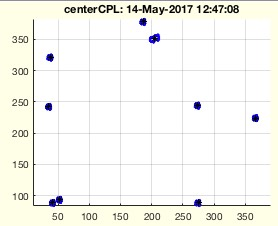% centerCPL(CPL) - returns the center of all contours of a CPL% (by Tim Lueth, VLFL-Lib, 2017-MAI-14 as class: CLOSED POLYGON LISTS)% % This fnctn helps to find fiducial marker center in xray images (Status % of: 2017-05-14)% % Introduced first in SolidGeometry 3.8% % See also: centerPL, CPLcontourc% % PL=centerCPL(CPL)% === INPUT PARAMETERS ===% CPL: Closed Polygon list with several PL's% === OUTPUT RESULTS ======% PL: List of center of CPL using centerPL% % EXAMPLE: % VL=50*rand(10,3)-25; % I=imageofVLprojection(VL,[100 100],[0 -100 0],[0 100 0],4);% CPL=CPLcontourc(double(I),1);% centerCPL(CPL)% % See also: centerPL, CPLcontourc%
###### VLofimsegthres(I)- returns a VL of segmented points within an image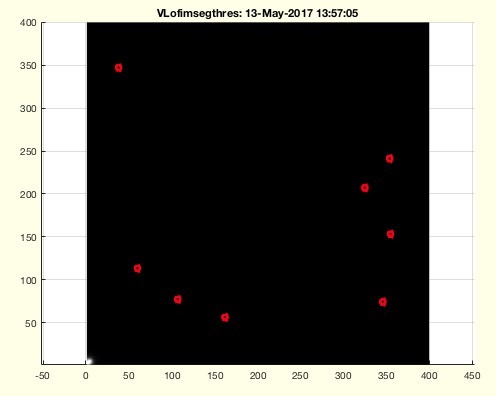% VLofimsegthres(I) - returns a VL of segmented points within an image% (by Tim Lueth, VLFL-Lib, 2017-MAI-12 as class: ANALYTICAL GEOMETRY)% % This fnctn helps to find the center of features islands within an image % (Status of: 2017-05-14)% % Introduced first in SolidGeometry 3.8% % [VLC,VLs,VL,S]=VLofimsegthres(I)% === INPUT PARAMETERS ===% I: Logical Image% === OUTPUT RESULTS ======% VLC: Vertex List of centers% VLs: size of features% VL: All feature poits% S: index list for features% % EXAMPLE: % VL=50*rand(10,3)-25;% I=imageofVLprojection(VL,[100 100],[0 -100 0],[0 100 0],4);% VLofimsegthres(I<200);%
###### Tofcam(pc,pt,cup)- returns HT matrix of camera and target for a given camera point, target point, and camera up vector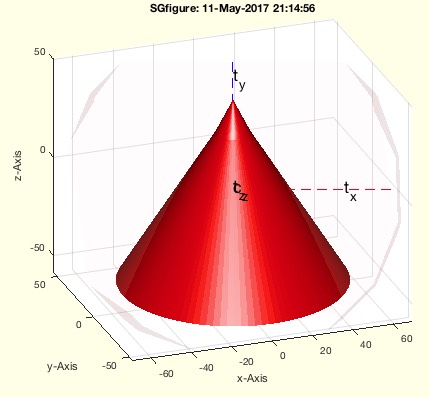% Tofcam(pc,pt,cup) - returns HT matrix of camera and target for a given camera point, target point, and camera up vector% (by Tim Lueth, VLFL-Lib, 2017-MAI-11 as class: USER INTERFACE)% % Introduced first in SolidGeometry 3.8% % See also: Tofgca% % [T,Tt,va,ax]=Tofcam(pc,pt,cup)% === INPUT PARAMETERS ===% pc: point of camera; default is campos% pt: point of target; default is camtarget% cup: cup direction ; rough; default is camup% === OUTPUT RESULTS ======% T: Transformation of camera% Tt: Transformation of target% va: current camera angle% ax: current axis values% % EXAMPLE: % SGfigure; SGplot(SGsample(1)); pause; Tofcam% % See also: Tofgca%
###### imageofVLprojection(VL,isize,pc,pt,pixnum,cup)- returns an image of a VL projection using the matlab camera view concept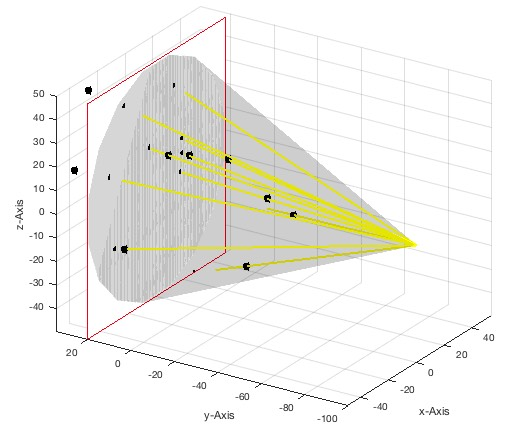% imageofVLprojection(VL,isize,pc,pt,pixnum,cup) - returns an image of a VL projection using the matlab camera view concept% (by Tim Lueth, VLFL-Lib, 2017-MAI-11 as class: ANALYTICAL GEOMETRY)% % To get a real projection image using the graphics camera parameter it % is absolutely necessary to switch to perspective mode first (this is % part of this fnctn) before taking a snapshot (getframe);% >> set(gca,'Projection','perspective');% The image size in mm defines the opening angle: 2*tan(alpha)=x/2 / % norm(pc-pt)% The angle alpha is the complete opening angle, same for both dimensions.% Therefor the target screen height is x = 2 * tan (alpha/2) * % norm(pc-pt) (Status of: 2017-05-12)% % Introduced first in SolidGeometry 3.8% % See also: imwarpT% % [I,Ic,pixs,Tc,Tt,C]=imageofVLprojection([VL,isize,pc,pt,pixnum,cup])% === INPUT PARAMETERS ===% VL: Vertex list; default is a square for testing% isize: image size [x y] centered; this defines the view angle% pc: position of camera; default is [0 +100 0];% pt: position of screen; default is [0 -100 0];% pixnum: pixels of image; default is isize;% cup: cup vector; default is [1 0 0]% === OUTPUT RESULTS ======% I: Grey scale matrix [0..1]% Ic: Color Matrix struct I.cdata% pixs: pixel size in mm; scaling% Tc: HT matrix of camera position% Tt: HT matrix of target position% C: SG of the perspective cone in space% % EXAMPLE: % imageofVLprojection('',[200 200],[0 -400 0],[0 100 0],1); % Original % imageofVLprojection('',[200 200],[0 -400 0],[0 100 0],2); % Dots smaller% imageofVLprojection('',[200 200],[0 -400 0],[0 200 0],2); % Dots outward% imageofVLprojection('',[200 200],[0 -400 0],[0 600 0],2); % Dots out% imageofVLprojection(100*rand(10,3)-50,[200 200],[0 -400 0],[0 600 0]);% % See also: imwarpT%
###### imwarpT(I,T,scal,cent,fc)- warps an image related to a give transformation matrix% imwarpT(I,T,scal,cent,fc) - warps an image related to a give transformation matrix% (by Tim Lueth, VLFL-Lib, 2017-MAI-10 as class: USER INTERFACE)% % Introduced first in SolidGeometry 3.8% % See also: VLwarpgrid, warp, imwarp, imageofVLprojection% % h=imwarpT(I,[T,scal,cent,fc])% === INPUT PARAMETERS ===% I: image or image size [r c]% T: Transformation matrix% scal: scaling factor% cent: true if centered; default is false% fc: frame color such as 'b-'; default is '';% === OUTPUT RESULTS ======% h: handle to image % % EXAMPLE: % I=imread('cameraman.tif');% imwarpT(I,TofR(rot(pi/6,pi/6,pi/6))); % imwarpT(I,TofR(rot(pi/6,pi/3,pi/6)));% SGfigure; imwarpT(I,TofR(rot(pi/6,pi/6,pi/6)),[0.1 0.1],true);% SGfigure; imwarpT(I,eye(4),[0.1 0.1],true,'b-'); show% % See also: VLwarpgrid, warp, imwarp, imageofVLprojection%
###### VLwarpgrid(siz,T,scal)- creates a meshgrid for warping an image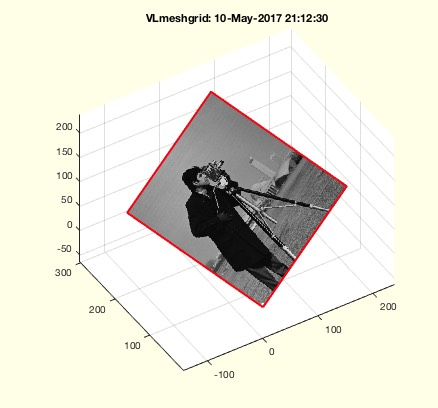% VLwarpgrid(siz,T,scal) - creates a meshgrid for warping an image% (by Tim Lueth, VLFL-Lib, 2017-MAI-10 as class: USER INTERFACE)% % See also: imwarpT% % [VL,XL,YL,ZL]=VLwarpgrid([siz,T,scal])% === INPUT PARAMETERS ===% siz: image or image size [r c]% T: Transformation matrix% scal: scaling factor% === OUTPUT RESULTS ======% VL: Vertex list% XL: warp grid X% YL: warp grid Y% ZL: warp grid Z% % EXAMPLE: % I=imread('cameraman.tif');% VLwarpgrid(I,TofR(rot(pi/6,0,0)));;%
###### PLofVLprojection(VL,pc,pt,cup,sc,isize)- returns image point on a target screen% PLofVLprojection(VL,pc,pt,cup,sc,isize) - returns image point on a target screen% (by Tim Lueth, VLFL-Lib, 2017-MAI-07 as class: ANALYTICAL GEOMETRY)% % Fnctn to simulate a c-arm or central projection to a flat screen % (Status of: 2017-05-09)% % See also: TofcamVLPL% % [PL,T,VLT,Tc]=PLofVLprojection([VL,pc,pt,cup,sc,isize])% === INPUT PARAMETERS ===% VL: Vertex list% pc: camera center% pt: target image origin% cup: camera up center; default is [0 0 1]% sc: image scaling (pixel size); default is [1 1]% isize: image size; default is 512 512% === OUTPUT RESULTS ======% PL: Point List in image coordinate system% T: T matrix of image coordinate system% VLT: Position of the projektion points in VL's system% Tc: T matrix of camera coordinate system% % EXAMPLE: % PLofVLprojection(VLsample(7),[0 -50 0],[0 20 30],[1 0 0])% PLofVLprojection(VLsample(7),[0 -50 0],[0 20 30],[0 0 1])% VL=10*rand(10,3), PLofVLprojection(VL)%
###### TofVLprojection()- returns the HT matrix of camera based on a VL and Projection image PL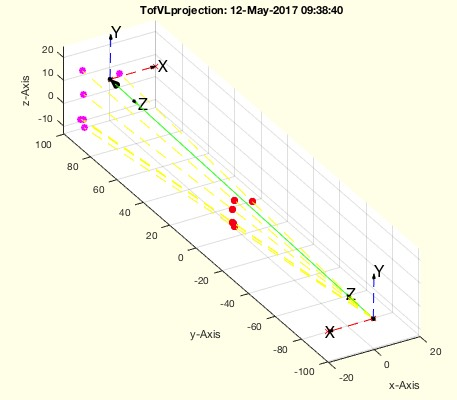% TofVLprojection() - returns the HT matrix of camera based on a VL and Projection image PL% (by Tim Lueth, VLFL-Lib, 2017-MAI-06 as class: ANALYTICAL GEOMETRY)% % =======================================================================% OBSOLETE (2017-05-12) - USE FAMILY 'TofcamVLPL' INSTEAD% =======================================================================% % exactly the same as TofcamVLPL (Status of: 2017-05-07)% % [T,K,s,azxz]=TofVLprojection([])% === OUTPUT RESULTS ======% T: % K: % s: % azxz: %
###### Tofgca- returns the HT matrix of the current camera position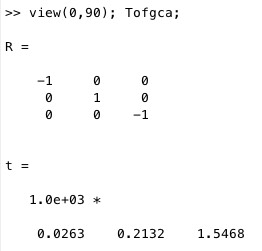% Tofgca - returns the HT matrix of the current camera position% (by Tim Lueth, VLFL-Lib, 2017-MAI-02 as class: ANALYTICAL GEOMETRY)% % Tofgca returns the current axis's camera values as HT Matrix% Tofcam returns either for given or the current axis's camera values as % HT Matrix (Status of: 2017-05-11)% % See also: showcam, Tofcam% % [T,Tt,va,ax]=Tofgca% === OUTPUT RESULTS ======% T: HT matrix of camera coordinate system% Tt: HT of target coordinate system% va: current camera angle% ax: current camera axis% % EXAMPLE: Just try% SGfigure; SGplot(SGsample(1)); pause (1); Tofgca; pause(1); axis tight; % view(-30,30)%
###### showcam- draws a copy of the current figure including the current camera situation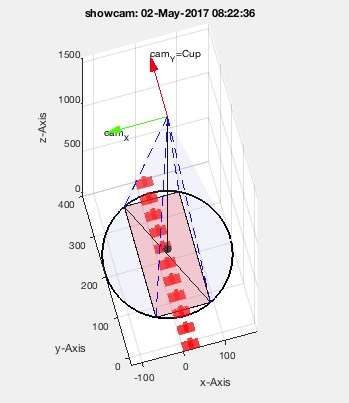% showcam - draws a copy of the current figure including the current camera situation % (by Tim Lueth, VLFL-Lib, 2017-MAI-01 as class: VISUALIZATION)% % See also: Tofgca, camset, campos, camplot% % showcam% % EXAMPLE: % SGfigure; SGmodelOR(1)% showcam%
###### exp_2017_05_01- EXPERIMENT that visuaize the current projection situation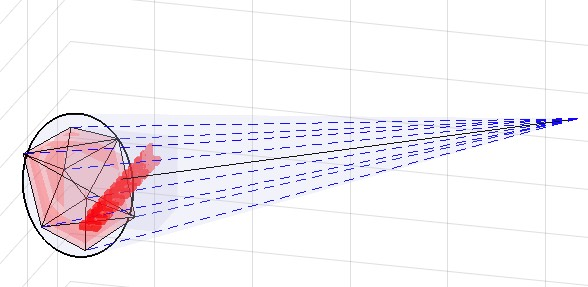% exp_2017_05_01 - EXPERIMENT that visuaize the current projection situation% (by Tim Lueth, VLFL-Lib, 2017-MAI-01 as class: USER INTERFACE)% % Final fnctn called: showcam (Status of: 2017-05-01)% % See also: camset, camplot% % exp_2017_05_01%
###### SGof2CPLsz(CPA,CPB,z,stype,ctype)- returns a solid defined by 2 contours and height% SGof2CPLsz(CPA,CPB,z,stype,ctype) - returns a solid defined by 2 contours and height% (by Tim Lueth, VLFL-Lib, 2017-APR-24 as class: SURFACES)% % This fnctn is the same as SGof2CPLz but supports more than one contour!% The optimal result depends on the expectations of the user. Consider % different options for stype carefully:% "number" of points, i.e. 1:nmax% "length" of contour, i.e. 0:sum(edge length)% "angle" of contour, i.e. 0:sum(abs(edge angle))% "center" of contour, i.e. 0:360 degree% also the starting point ctype ('rot' or 'min');% Quite optimal procedure to find the walls between two planar contours % without adding new points. The number of faces is na+nb. % The resulting vertex list SG: % SG.VL=[VLaddz(CPA,0);VLaddz(CPB,z)];% SG.FL=[FLA;FLB;FLW];% (Status of: 2017-06-12)% % Introduced first in SolidGeometry 3.8% % See also: SGof2SGT, SGof2T, SGof2CVL, SGof2CPLz, SGofCPLzchamfer, % SGof2CPLzheurist, SGof2CPLzbranch% % [SG,FLW,FLA,FLB]=SGof2CPLsz([CPA,CPB,z,stype,ctype])% === INPUT PARAMETERS ===% CPA: ONE bottom Contour% CPB: ONE top Contour% z: height of the solid% stype: "number", "length", "angle", "center"; default is "length"% ctype: "rot" or "miny"; default is "rot"% === OUTPUT RESULTS ======% SG: Solid Geoemtry; VL=[CPA;CPB]% FLW: Face list of Wall% FLA: Face list of CPA% FLB: Face list of CPB% % EXAMPLE: % CPA=[PLcircle(5.1,16);NaN NaN; PLcircle(2,4)]; % CPB=[PLstar(5,16,[],[],[],0.5);NaN NaN; PLcircle(2)]; % SGof2CPLsz(CPA,CPB,5)% % See also: SGof2SGT, SGof2T, SGof2CVL, SGof2CPLz, SGofCPLzchamfer, % SGof2CPLzheurist, SGof2CPLzbranch%
###### exp_2017_04_24- EXPERIMENT to create drawing templates with prismatic angles =>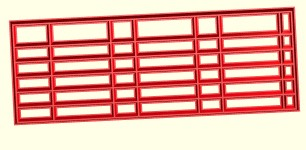% exp_2017_04_24 - EXPERIMENT to create drawing templates with prismatic angles => % (by Tim Lueth, VLFL-Lib, 2017-APR-24 as class: EXPERIMENTS)% % EXPERIMENT to create a fnctn for drawing templates (Zeichen schablone)% Resulting fnctns are% 1. SGof2CPLsz% (Status of: 2017-04-24)% % exp_2017_04_24%
###### BBofCPL(CPL)- returns the bounding box of a CPL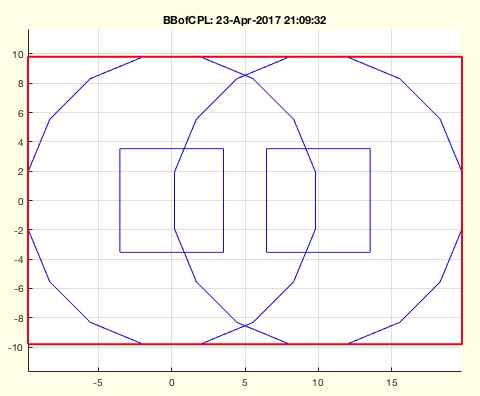% BBofCPL(CPL) - returns the bounding box of a CPL% (by Tim Lueth, VLFL-Lib, 2017-APR-23 as class: CLOSED POLYGON LISTS)% % See also: CPLofBB, BBofVL, BBofSG% % bb=BBofCPL(CPL)% === INPUT PARAMETERS ===% CPL: Closed Polygon Line% === OUTPUT RESULTS ======% bb: bb [xmin xmax ymin ymax]%
###### CPLofmeshgrid(X,Y)- returns two grid line templates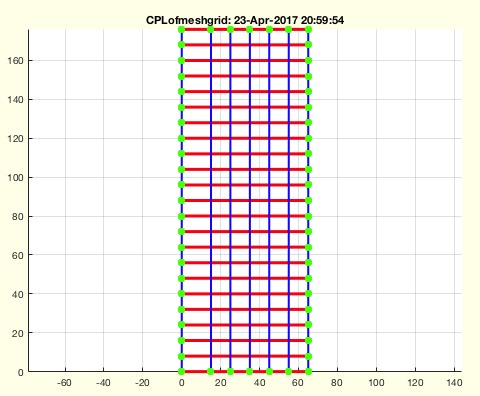% CPLofmeshgrid(X,Y) - returns two grid line templates% (by Tim Lueth, VLFL-Lib, 2017-APR-23 as class: CLOSED POLYGON LISTS)% % See also: CPLof% % [CPL,PLX,PLY,PL]=CPLofmeshgrid(X,Y)% === INPUT PARAMETERS ===% X: List of X values% Y: List of Y values% === OUTPUT RESULTS ======% CPL: Complete list of lines% PLX: Only X lines% PLY: Only Y lines% PL: Point List (unique)% % EXAMPLE: % CPLtemplateofCPL(CPLofmeshgrid([0 15 25 35 45 55 65],0:8:8*22),1,true);% SGdrawingtemplateofCPL(CPLofmeshgrid([0 15 25 35 45 55 65],8*[0 1 6 8 % 13 15 20 23]),1)% SGdrawingtemplateofCPL(B,1)%
###### CPLunitesorted(CPL)- returns sorted and added closed polygons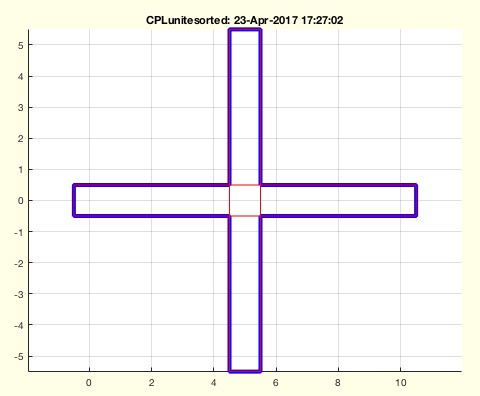% CPLunitesorted(CPL) - returns sorted and added closed polygons% (by Tim Lueth, VLFL-Lib, 2017-APR-23 as class: CLOSED POLYGON LISTS)% % ..have the impression to have implemented this fnctn already several % times in different manner.. (Status of: 2017-04-23)% % See also: CPLsortinout, CPLpolybool% % CPLN=CPLunitesorted(CPL)% === INPUT PARAMETERS ===% CPL: Closed Polygon Line% === OUTPUT RESULTS ======% CPLN: systematically ordered and Boolean operated CPL% % EXAMPLE: % A=[0 0; 10 0;NaN NaN;5 -5; 5 +5]% CPLunitesorted(CPLtemplateofCPL(A))%
###### CPLtemplateofCPL(CPL,pen,un)- returns a template for a given CPL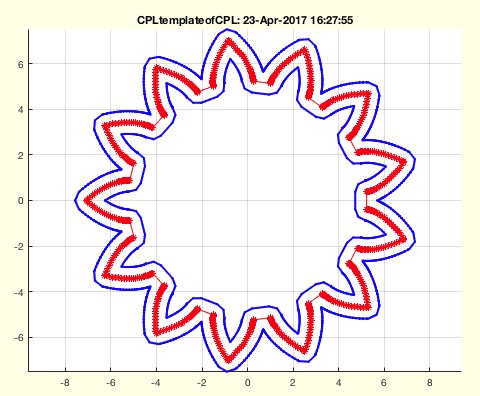% CPLtemplateofCPL(CPL,pen,un) - returns a template for a given CPL% (by Tim Lueth, VLFL-Lib, 2017-APR-23 as class: CLOSED POLYGON LISTS)% % Attention: This fnctn distinguishs between open and closed PL / CPLs % for obvious reasons. Threfor close a PL such as PLcircle or PLstar by % CPLofPL. (Status of: 2017-04-23)% % Introduced first in SolidGeometry 3.8% % See also: CPLgrow, PLgrow% % CPN=CPLtemplateofCPL(CPL,[pen,un])% === INPUT PARAMETERS ===% CPL: CPL separated by NaN NaN% pen: diameter of template% un: if true; contours are united; default is false;% === OUTPUT RESULTS ======% CPN: % % EXAMPLE: % A=[0 0; 10 0;NaN NaN;5 -5; 5 +5]; CPLtemplateofCPL(A,2,false)% A=[0 0; 10 0;NaN NaN;5 -5; 5 +5]; CPLtemplateofCPL(A,2,true)% CPLtemplateofCPL(PLsample(1))% CPLtemplateofCPL(PLsample(2))% CPLtemplateofCPL(PLsample(11),12)% CPLtemplateofCPL(CPLoftext('s'))% CPLtemplateofCPL(PLgearDIN(1,13))% % See also: CPLgrow, PLgrow%
###### PLsample(n)- returns a 2D PL list similar to VLsample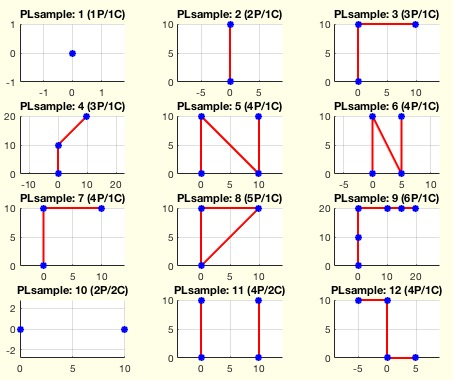% PLsample(n) - returns a 2D PL list similar to VLsample% (by Tim Lueth, VLFL-Lib, 2017-APR-23 as class: ANALYTICAL GEOMETRY)% % For many fnctns it is helpful to have a sample point list (Status of: % 2017-07-16)% % Introduced first in SolidGeometry 3.8% % See also: CPLsample, VLsample, SGsample, CPLoftext, VLFLsample% % PL=PLsample([n])% === INPUT PARAMETERS ===% n: sample of Point List% === OUTPUT RESULTS ======% PL: returns PL if n>0% % See also: CPLsample, VLsample, SGsample, CPLoftext, VLFLsample%
###### SGdrawingtemplateofCPL(CPLU,pen,unt,wall,height,dmin,lnk,top)- Creates a 3D printable drawing template - slow but helpful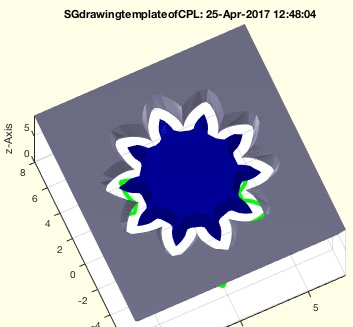% SGdrawingtemplateofCPL(CPLU,pen,unt,wall,height,dmin,lnk,top) - Creates a 3D printable drawing template - slow but helpful% (by Tim Lueth, VLFL-Lib, 2017-APR-23 as class: MODELING PROCEDURES)% % When creating templates from a line drawing, a contour is moved % outwards and inwards: CPLgrow. This can lead to self-overlaps of % contours. Accordingly, the out-contour of the grown contour and the % inner contour of the fringed contour must be used: CPLsortinout. The % fnctn is: CPLtemplateofCPL. Insulated contours are then connected: % CPLunitesorted. Overlapping contours are preserved by auxiliary points. % (Status of: 2017-04-25)% % Introduced first in SolidGeometry 3.8% % See also: CPLtemplateofCPL% % SG=SGdrawingtemplateofCPL(CPLU,[pen,unt,wall,height,dmin,lnk,top])% === INPUT PARAMETERS ===% CPLU: Linienzeichnung% pen: pen diameter% unt: if true; crossings are united; default is false% wall: wall thickness; default is 1.5mm% height: height of template; default is 1.5mm% dmin: diameter to support crossing points% lnk: if true, solids are connected; default is false% top: upper pen diameter; default is width;% === OUTPUT RESULTS ======% SG: SG of a drawing template% % EXAMPLE: % A=[0 0; 10 0;NaN NaN;5 -5; 5 +5]; SGdrawingtemplateofCPL(A,1,false);% A=[0 0; 10 0;NaN NaN;5 -5; 5 +5]; SGdrawingtemplateofCPL(A,1,true);% SGdrawingtemplateofCPL([0 0; 10 0])% SGdrawingtemplateofCPL(PLgearDIN(1,10))% % See also: CPLtemplateofCPL%
###### PLsplitpointsofCPL(CPL)- returns splitpoints created by delaunaytriangulation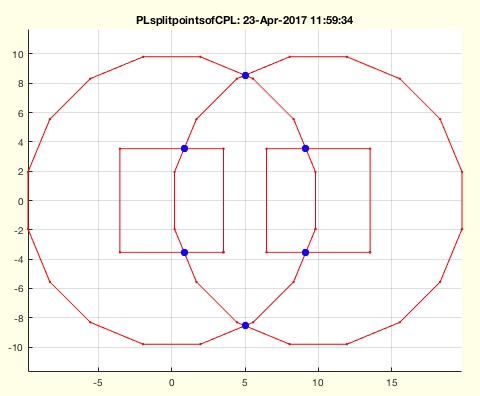% PLsplitpointsofCPL(CPL) - returns splitpoints created by delaunaytriangulation% (by Tim Lueth, VLFL-Lib, 2017-APR-23 as class: CLOSED POLYGON LISTS)% % Introduced first in SolidGeometry 3.8% % See also: CPLsplitpoints% % SPL=PLsplitpointsofCPL(CPL)% === INPUT PARAMETERS ===% CPL: CPL separated by NaN% === OUTPUT RESULTS ======% SPL: List of splitpoints created by delaunaytriangulation% % EXAMPLE: % PLsplitpointsofCPL(CPLsample(16))% % See also: CPLsplitpoints%
###### SGcopyVL(SG,VL,fuse)- returns a cell list of copies of SG an positions of VL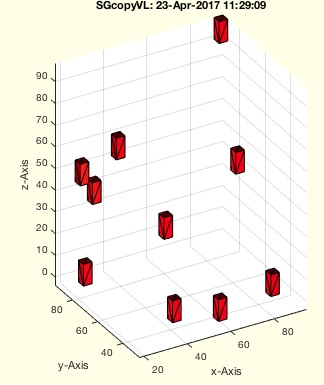% SGcopyVL(SG,VL,fuse) - returns a cell list of copies of SG an positions of VL% (by Tim Lueth, VLFL-Lib, 2017-APR-23 as class: SURFACES)% % Introduced first in SolidGeometry 3.8% % See also: SGarrangeSG, SGcopyrotZ% % SGC=SGcopyVL(SG,VL,[fuse])% === INPUT PARAMETERS ===% SG: Solid Geometry% VL: Coordiantes for creating a copy of SG% fuse: if true; SG is struct not cell; default is true% === OUTPUT RESULTS ======% SGC: cell list of SG% % EXAMPLE: Create copies at ten random positions% SGcopyVL(SGbox([4,4,10]),100*rand(10,3))% % See also: SGarrangeSG, SGcopyrotZ%
###### lastwarnTL- returns information on the last warnings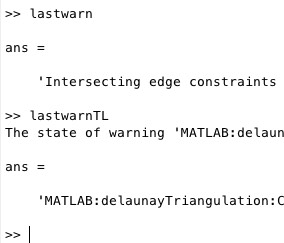% lastwarnTL - returns information on the last warnings% (by Tim Lueth, VLFL-Lib, 2017-APR-23 as class: AUXILIARY PROCEDURES)% % For a programmer, who wants to know which warning should be switched % off, the sequence of lastwarn is may not optimal; should be flipped % (Status of: 2017-07-15)% % Introduced first in SolidGeometry 3.8% % See also: lastwarnoff, lastwarn% % [b,a]=lastwarnTL% === OUTPUT RESULTS ======% b: LASTID% a: LASTMSG% % EXAMPLE: % lastwarnTL% warning('off','last');% % % See also: lastwarnoff, lastwarn%
###### ginput2Dcircle(gr,ax)- returns by user interaction center and radius of a circle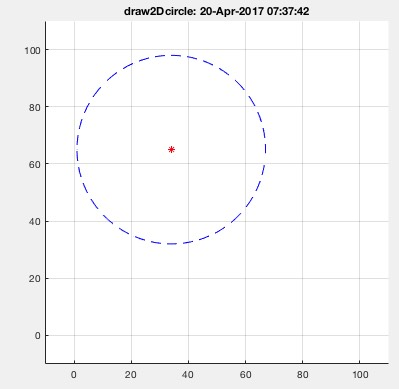% ginput2Dcircle(gr,ax) - returns by user interaction center and radius of a circle% (by Tim Lueth, VLFL-Lib, 2017-APR-20 as class: USER INTERFACE)% % Introduced first in SolidGeometry 3.8% % [cc,cr,h]=ginput2Dcircle([gr,ax])% === INPUT PARAMETERS ===% gr: grid resolution; default is 1% ax: axis of the screen; default is % === OUTPUT RESULTS ======% cc: center of circle% cr: radius of circle% h: %
###### SGofpatch(gh,fuse)- returns a SG from a patch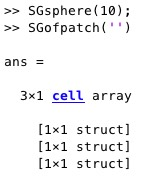% SGofpatch(gh,fuse) - returns a SG from a patch% (by Tim Lueth, VLFL-Lib, 2017-APR-19 as class: SURFACES)% % See also: patchofSG, SGplot, reducepatch, reducepatchSG% % SG=SGofpatch([gh,fuse])% === INPUT PARAMETERS ===% gh: handle to patch; default is patchofgca% fuse: if true; create only one SG; default is false;% === OUTPUT RESULTS ======% SG: Solid Geoemtry; single or cells%
###### patchofSG(SG,realp)- creates a patch struct of a solid geometry% patchofSG(SG,realp) - creates a patch struct of a solid geometry% (by Tim Lueth, VLFL-Lib, 2017-APR-19 as class: USER INTERFACE)% % Matlab has different data models for the handling of surface models:% patch, triangulation, delaunaytriangulation, poly2fv etc.% SUPPORTS also with cells of solids an returns a list% ATTENTION: A patch is only valid if the figure is not closed. So in % case that you want to use a patch for surface reduction, copy it % afterwards into a SG using 'SGofpatch'; It is similar to SGplot (Status % of: 2017-06-17)% % See also: SGofpatch, SGplot, SGreduceVLFL% % h=patchofSG(SG,[realp])% === INPUT PARAMETERS ===% SG: Soldid Geometry; % realp: true=real patch; default is false% === OUTPUT RESULTS ======% h: handle to patch struct or patch class% % EXAMPLE: % a=patchofSG(SGsample(17))% a=patchofSG(SGsample(17),true)%
###### patchofgca(gh)- returns all patches of the current graphics axis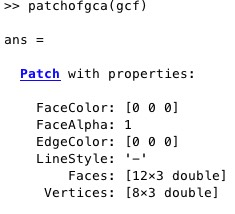% patchofgca(gh) - returns all patches of the current graphics axis% (by Tim Lueth, VLFL-Lib, 2017-APR-19 as class: USER INTERFACE)% % simple macro: h=findall(gh,'type','patch'); (Status of: 2017-04-19)% % See also: SGofpatch, patchofSG, SGplot, reducepatch% % h=patchofgca([gh])% === INPUT PARAMETERS ===% gh: gca or gcf; default gca% === OUTPUT RESULTS ======% h: handle / handle array%
###### reducepatchSG(h,f)- reduces either one or all patches of the current axis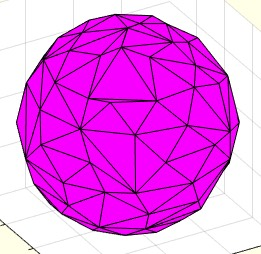% reducepatchSG(h,f) - reduces either one or all patches of the current axis% (by Tim Lueth, VLFL-Lib, 2017-APR-18 as class: SURFACES)% % =======================================================================% OBSOLETE (2017-06-17) - USE FAMILY 'SGreduceVLFL' INSTEAD% =======================================================================% % exactly the same as reducepatch, but works with several path arrays and % has default values (Status of: 2017-06-16)% % See also: [ SGreduceVLFL ] ; reducepatch, daspect% % h=reducepatchSG([h,f])% === INPUT PARAMETERS ===% h: handle; default is findall(gca,'type','patch') or SG% f: reduction factor; default is 0.5% === OUTPUT RESULTS ======% h: handle or list of handle of gca or SG% % EXAMPLE: % SGsphere(10); reducepatchSG('',0.1); show% SGsphere(10); reducepatchSG('',500); show% SGbox([30,20,10]); pause(1); reducepatchSG('',0.5); show%
###### SGofVMisosurface(V,vs,cl)- returns a surface model by the marching cubes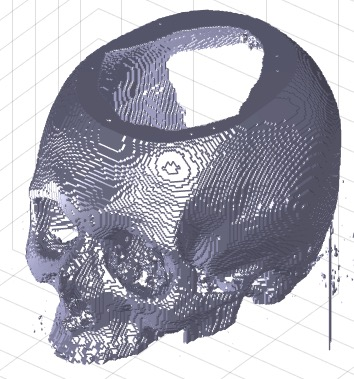% SGofVMisosurface(V,vs,cl) - returns a surface model by the marching cubes% (by Tim Lueth, VLFL-Lib, 2017-APR-18 as class: VOXELS)% % This fnctn is an original Matlab fnctn just a little bit modified to % close surfaces. The fnctn is very similar of SGofVMmarchcube but % definitely slower. Main problem is the unclear method that is % implemented by Matlab. (Status of: 2017-04-18)% % Introduced first in SolidGeometry 3.8% % See also: VLFLofVMdelaunay, VLFLofVMmarchcube, TR3ofVM, % SGofVMmarchcube, SGofVMdelaunay% % SG=SGofVMisosurface(V,[vs,cl])% === INPUT PARAMETERS ===% V: Logical Volume Model% vs: voxel size, optional% cl: closes open facets by adding empty planes; default is true% === OUTPUT RESULTS ======% SG: Vertex list% % EXAMPLE: [V,vs]=VMreaddicomdir('AIM_DICOMFILES');% [a,as]=VMresize(V,[0.5 0.5 0.5],vs);% SG=SGofVMisosurface(a>1400,as);% SGcut(SG,40);% c=CPLofSGslice3(SG,20);% PLFLofCPLdelaunay(c);% PLFLofCPLpoly(c)% % See also: VLFLofVMdelaunay, VLFLofVMmarchcube, TR3ofVM, % SGofVMmarchcube, SGofVMdelaunay%
###### isatleastVer(name,TB)- checks the minimal version/release number of matlab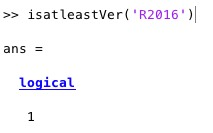% isatleastVer(name,TB) - checks the minimal version/release number of matlab% (by Tim Lueth, VLFL-Lib, 2017-APR-18 as class: AUXILIARY PROCEDURES)% % Introduced first in SolidGeometry 3.8% % See also: verTL% % is=isatleastVer(name,[TB])% === INPUT PARAMETERS ===% name: Release name 'R2017a'% TB: Toolbox name ;default is 'Matlab'% === OUTPUT RESULTS ======% is: true or false% % EXAMPLE: % isatleastVer('R2017b')% isatleastVer('R2017a')% % See also: verTL%
###### verTL(name)- returns the currently used Matlab Release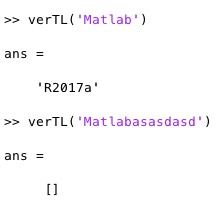% verTL(name) - returns the currently used Matlab Release% (by Tim Lueth, VLFL-Lib, 2017-APR-18 as class: AUXILIARY PROCEDURES)% % More simple to use than 'ver' (Status of: 2017-07-07)% % Introduced first in SolidGeometry 3.8% % See also: ver, isatleastVer% % v=verTL(name)% === INPUT PARAMETERS ===% name: Tool to search for% === OUTPUT RESULTS ======% v: RElease such as 'R2017a'; empty if missing; % % EXAMPLE: % ver% verTL('Matlab')% verTL('Matlabasasdasd')% verTL('Mapping Toolbox')% % % See also: ver, isatleastVer%
###### VMwindowplot(V,cax,minv,maxv)- plots a voxel model using the window caxis function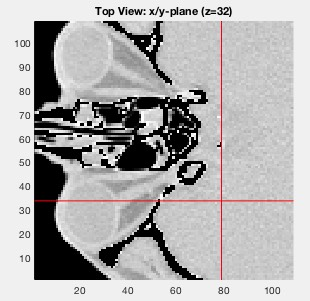% VMwindowplot(V,cax,minv,maxv) - plots a voxel model using the window caxis fnctn% (by Tim Lueth, VLFL-Lib, 2017-APR-12 as class: VOXELS)% % shows only the voxels within the specified intensity interval and voxel % intervall (Status of: 2017-04-17)% % See also: VMplot, VMcaxis% % VX=VMwindowplot(V,[cax,minv,maxv])% === INPUT PARAMETERS ===% V: Voxel model% cax: [cmin cmax];% minv: minimum voxel value; values <0 are precentage% maxv: maximum voxel value; values <0 are precentage% === OUTPUT RESULTS ======% VX: % % EXAMPLE: % VMwindowplot(V,[400 1200],0.2,0.7);% SGofVMmarchcube(VX)%
###### VMcaxis (cax)- adjustes the caxis of the VMplot diagram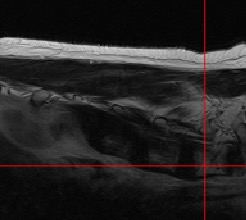% VMcaxis (cax) - adjustes the caxis of the VMplot diagram% (by Tim Lueth, VLFL-Lib, 2017-APR-12 as class: VOXELS)% % VMcaxis([cax])% === INPUT PARAMETERS ===% cax: [cmin cmax]; default is min and max of the current! surfaces%
###### numofstrtab(tok,strtab)- returns a row number of a cell list of strings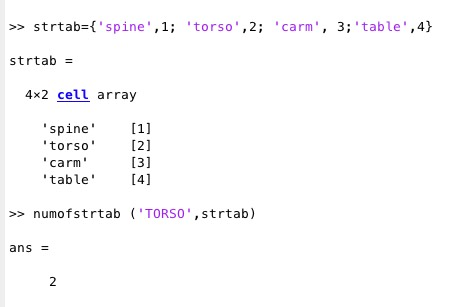% numofstrtab(tok,strtab) - returns a row number of a cell list of strings% (by Tim Lueth, VLFL-Lib, 2017-APR-09 as class: AUXILIARY PROCEDURES)% % See also: SGmodelOR% % nr=numofstrtab(tok,strtab)% === INPUT PARAMETERS ===% tok: string to compare with;% strtab: cell list; frist col = string% === OUTPUT RESULTS ======% nr: cell list row, col 2: end% % EXAMPLE: % strtab={'spine',1; 'torso',2; 'carm', 3;'table',4};% numofstrtab ('TORSO',strtab);%
###### SGmodelOR(part)- returns solid model of OR device models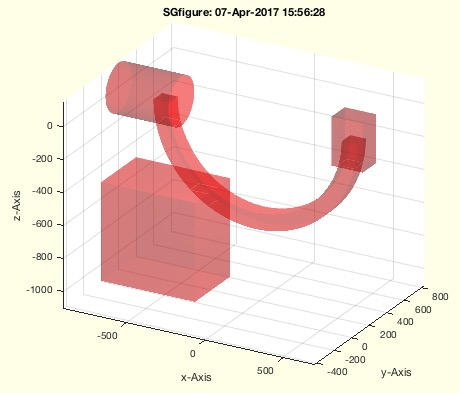% SGmodelOR(part) - returns solid model of OR device models% (by Tim Lueth, VLFL-Lib, 2017-APR-07 as class: SURFACES)% % The following (Status of: 2017-04-17)% % Introduced first in SolidGeometry 3.8% % See also: SGsample, CPLsample, VLSample% % SG=SGmodelOR(part)% === INPUT PARAMETERS ===% part: % === OUTPUT RESULTS ======% SG: Solid geometry of part% % See also: SGsample, CPLsample, VLSample%
###### CELofEL(EL)- returns a corrected succeding EL for a single contour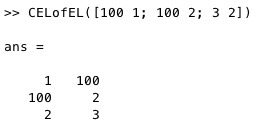% CELofEL(EL) - returns a corrected succeding EL for a single contour% (by Tim Lueth, VLFL-Lib, 2017-APR-07 as class: EDGE LISTS)% % branches are not alloweed, i.e. % - two terminals and m links % - or n links (Status of: 2017-04-07)% % See also: treeNodesofEL% % [CEL,CHN]=CELofEL(EL)% === INPUT PARAMETERS ===% EL: unsorted but correct edge list% === OUTPUT RESULTS ======% CEL: Sorted Edge list of Contour or Line of Nodes% CHN: Chain [n x 1] with nodes% % EXAMPLE: % CELofEL([100 1; 100 2; 3 2; 1 3])%
###### ELsort(EIL)- returns a increasing sorted edge list separated by NaN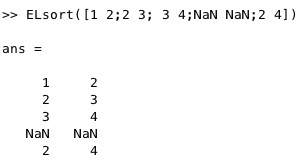% ELsort(EIL) - returns a increasing sorted edge list separated by NaN% (by Tim Lueth, VLFL-Lib, 2017-APR-06 as class: AUXILIARY PROCEDURES)% % Does replace sortEL. Branches are not allowed! Use % This fnctn is able to handle NaN separated Edge lists and orders them % upwards from frist to last and left to right. In addition the chains % are linked together% woNaN can be used ti remove the NaN NaN separators later. (Status of: % 2017-04-07)% % See also: ELunsort, ELuniqueofFL, CELofEL, woNaN% % EIL=ELsort(EIL)% === INPUT PARAMETERS ===% EIL: Edge List% === OUTPUT RESULTS ======% EIL: Edge List%
###### treeNodesofEL(EL)- returns the tree structure from a given edge list% treeNodesofEL(EL) - returns the tree structure from a given edge list% (by Tim Lueth, VLFL-Lib, 2017-APR-06 as class: EDGE LISTS)% % Fnctn removes lines with Nan first: In a list there are: % - single used points (Terminals)% - doubled used points (Links)% - three or more times used points (Branches)% (Status of: 2017-04-07)% % See also: CELofEL% % [vi,vc,bi,ti,li]=treeNodesofEL(EL)% === INPUT PARAMETERS ===% EL: Edge list% === OUTPUT RESULTS ======% vi: list of vertices [vi n]% vc: vertes use counter [bi ti li]% bi: branch index% ti: terminal index% li: link index% % EXAMPLE: % A=SGbox([30,20,10]); EL=FEofSG(A); treeNodesofEL(EL)%
###### VLremsuccident(VL)- removes succeding identical rows in a list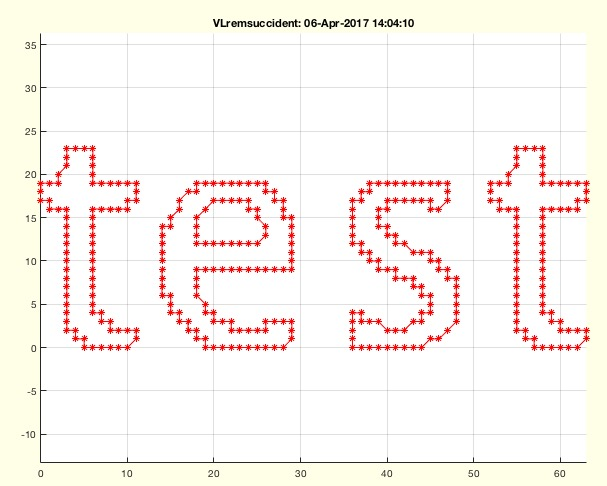% VLremsuccident(VL) - removes succeding identical rows in a list% (by Tim Lueth, VLFL-Lib, 2017-APR-06 as class: AUXILIARY PROCEDURES)% % Fnctn is able to handle doubled nan too! It was designed for open PL % ways returns a PL/VL since last and frist point are succeeding points % and the first remains. (Status of: 2017-04-07)% % Introduced first in SolidGeometry 3.8% % See also: CVLremstraight% % [SVL,ii]=VLremsuccident(VL)% === INPUT PARAMETERS ===% VL: list of numeric rows% === OUTPUT RESULTS ======% SVL: decimated list% ii: removed indices in original list% % EXAMPLE: % CPL=roundn(CPLaddauxpoints(CPLoftext('test'),1),1)% VLremsuccident(CPL)% % See also: CVLremstraight%
###### CVLremstraight (CVL,de,al)- returns straight points on a line depending on distance and agle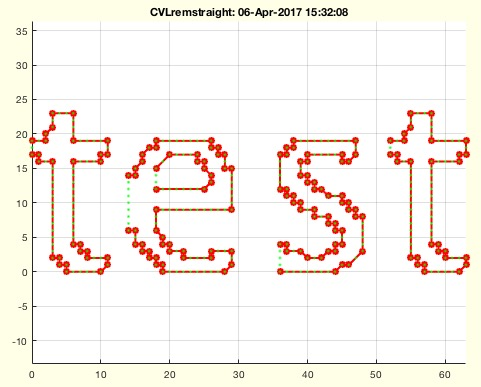% CVLremstraight (CVL,de,al) - returns straight points on a line depending on distance and agle% (by Tim Lueth, VLFL-Lib, 2017-APR-06 as class: ANALYTICAL GEOMETRY)% % able to handel CPL% checks identical points (1),(end)% removes identical succeeding points% removes points neadby % removes angle points less than one degree (Status of: 2017-04-06)% % Introduced first in SolidGeometry 3.8% % See also: VLremsuccident% % CVLremstraight(CVL,[de,al])% === INPUT PARAMETERS ===% CVL: Contour verteex list% de: default is 1e-6;% al: default is 1 degree% % EXAMPLE: % CPL=roundn(CPLaddauxpoints(CPLoftext('test'),1),1)% CVLremstraight(CPL)% % See also: VLremsuccident%
###### FEofSG(SG,ang)- returns FE and optional VL of a solid geometry or of gca% FEofSG(SG,ang) - returns FE and optional VL of a solid geometry or of gca% (by Tim Lueth, VLFL-Lib, 2017-APR-06 as class: SURFACES)% % Introduced first in SolidGeometry 3.8% % See also: VLFLofgca, FEplot% % [FE,VL]=FEofSG([SG,ang])% === INPUT PARAMETERS ===% SG: if empty; VLFLofgca is used% ang: angle; default is 0.4 ~ pi/8% === OUTPUT RESULTS ======% FE: Feature edge list% VL: Vertex list for embedded SG% % EXAMPLE: % FEofSG(SGsample(3))% % See also: VLFLofgca, FEplot%
###### viewdimensioning(a,b,crossp,alpha)- changes the view parameter for an engineering view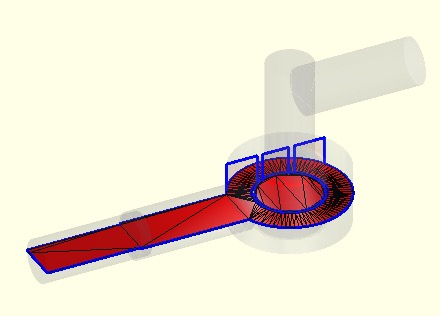% viewdimensioning(a,b,crossp,alpha) - changes the view parameter for an engineering view% (by Tim Lueth, VLFL-Lib, 2017-APR-05 as class: USER INTERFACE)% % This fnctn works similar to view but allows to add a crossing point to % see only a crossing plane;% Nevertheless such a crossing plane requires in fact a real slicing of % the sold at the right possition (Status of: 2017-04-17)% % Introduced first in SolidGeometry 3.8% % See also: view, PLdimensioning, CVLdimclassifier% % % [CPL,CVL,X]=viewdimensioning([a,b,crossp,alpha])% === INPUT PARAMETERS ===% a: view angle a% b: view angle b% crossp: optional crossing point / plane% alpha: alpha value % === OUTPUT RESULTS ======% CPL: Contour Point list x y 0% CVL: Contour vertex list x y z% X: Crossing Solid% % EXAMPLE: % SGfigure(SGsample(17)); CPL=viewdimensioning(0,0,[0 0 0]); % PLdimensioning(CPL);% SGfigure(SGsample(17)); CPL=viewdimensioning(-90,0,[0 0 0]); % PLdimensioning(CPL);% % close all; SGfigure(SGsample(17)); view(-30,30);% view(0,90)% viewdimensioning(0,-90,[0 0 15],0.1)% [a,b,c]=viewdimensioning(0,-90,[0 0 15],0.1);% SGplot(c); CVLplot(b,'b-',3);% % See also: view, PLdimensioning, CVLdimclassifier% %
###### CVLdimclassifier(OVL)- analyses contours and classifies different drawing elements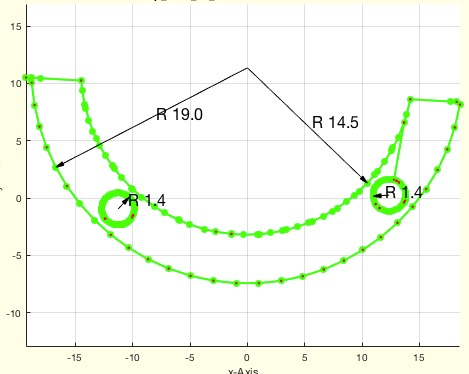% CVLdimclassifier(OVL) - analyses contours and classifies different drawing elements% (by Tim Lueth, VLFL-Lib, 2017-APR-04 as class: USER INTERFACE)% % This fnctn returns simple points that should be dimensioned. They do % not specify a contour. In addition the fnctn also returns a list of % radial centers and radius% [cx cy cz] is the center of a circle;% deg is the length of the circle% (Status of: 2017-06-25)% % Introduced first in SolidGeometry 3.8% % See also: PLdimensioning% % [DVL,RL,RIL,Rnv]=CVLdimclassifier(OVL)% === INPUT PARAMETERS ===% OVL: Contour vertex List% === OUTPUT RESULTS ======% DVL: List for dimensioning% RL: [cx cy cz Nr deg R vx vy vz]% RIL: Points % Rnv: List of normal vectors related to RL% % EXAMPLE: % CVLdimclassifier(PLradialEdges(CPLofPL(PLsquare(30,20)),4));% % % See also: PLdimensioning%
###### expname(pfix)- returns a filename with the current date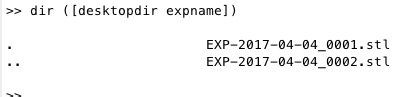% expname(pfix) - returns a filename with the current date% (by Tim Lueth, VLFL-Lib, 2017-APR-04 as class: FILE HANDLING)% % Introduced first in SolidGeometry 3.8% % See also: desktopdir, pcodedirTL, smbFilename, smbPSLibname% % fname=expname([pfix])% === INPUT PARAMETERS ===% pfix: prefix default is 'EXP-'% === OUTPUT RESULTS ======% fname: file or directory name% % EXAMPLE: % dir ([desktopdir expname])% % See also: desktopdir, pcodedirTL, smbFilename, smbPSLibname%
###### PLdimensioning(CPL)- plots a measurement ruler under a CPL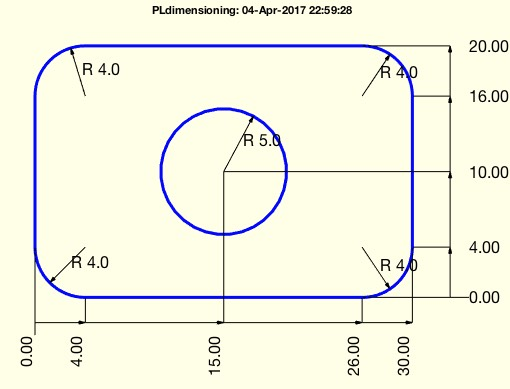% PLdimensioning(CPL) - plots a measurement ruler under a CPL% (by Tim Lueth, VLFL-Lib, 2017-APR-04 as class: USER INTERFACE)% % WORK in progress. Does support % - removal of points on straight lines% - holes and radial corners % (Status of: 2017-04-17)% % Introduced first in SolidGeometry 3.8% % See also: CPLsortinout, CVLdimclassifier, view, viewdimensioning% % h=PLdimensioning(CPL)% === INPUT PARAMETERS ===% CPL: CPL or PL% === OUTPUT RESULTS ======% h: handle to drawings% % EXAMPLE: % PLdimensioning(CPLsample(3));% PLdimensioning(CPLofPL(PLradialEdges(PLsquare(30,20),4)));% PLdimensioning(CPLofPL(PLradialEdges(CPLofPL(PLsquare(30,20)),4)));% PLdimensioning([CPLofPL(PLradialEdges(CPLofPL(PLsquare(30,20)),4));NaN % NaN;PLcircle(5)]);% % See also: CPLsortinout, CVLdimclassifier, view, viewdimensioning%
###### SGsurfacehistogram(B,si,be)- plots a surface area histogram of selected surfaces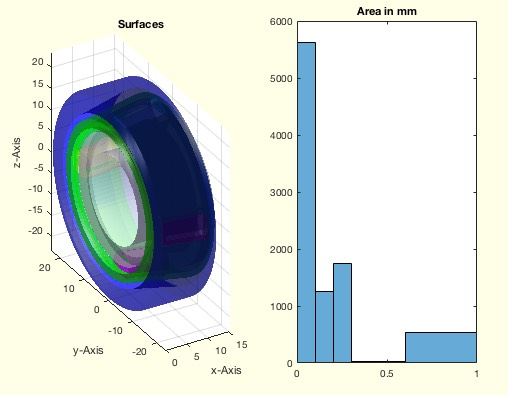% SGsurfacehistogram(B,si,be) - plots a surface area histogram of selected surfaces% (by Tim Lueth, VLFL-Lib, 2017-APR-04 as class: SURFACES)% % This fnctn is useful to analyze imported STL files generated by a CSG % modeler such as Catia.% Rounded edges or broken corners makes it difficult to analyze fnctnal % surface manually. This fnctn helps to get a feeling for the CSG-to-STL % conversion strategy. (Status of: 2017-04-04)% % Introduced first in SolidGeometry 3.8% % See also: SGsurfaceplot, SGsurfaces% % SGsurfacehistogram(B,[si,be])% === INPUT PARAMETERS ===% B: Solid Geoemtry% si: surface index wrt SGsurfaces; default ''% be: histogram bin edges; default is be=[0 0.1 0.2 0.3 0.4 0.5 0.6 1.0];% % % EXAMPLE: % SGsurfacehistogram(SGsample(17))% SGsurfacehistogram(SGsample(17),'',1:10)% % See also: SGsurfaceplot, SGsurfaces%
###### SGisconvex(SG)- returns whether a solid is convex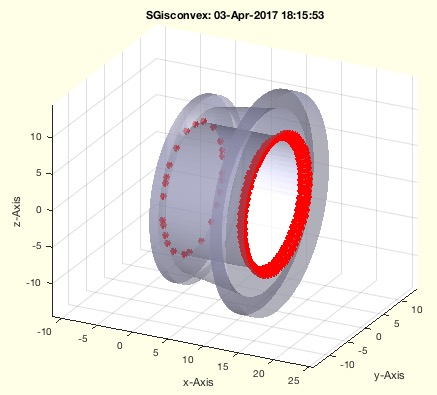% SGisconvex(SG) - returns whether a solid is convex% (by Tim Lueth & Felix Schoppa, VLFL-Lib, 2017-APR-03 as class: SURFACES)% % WORK IN PROGRESS (2017-04-03) % A solid is convex if all normal vectors of the vertices have the same % direction, i.e.if the angle between vector from center line to vertex % and verte normal vector is less than 90 degree (pi/2);% (Status of: 2017-04-03)% % c=SGisconvex(SG)% === INPUT PARAMETERS ===% SG: Solid Geometry% === OUTPUT RESULTS ======% c: true if convex, false if not%
###### SGsurfaces(SG,slct)- returns a cell list of separated CLOSED surfaces similar to SGseparate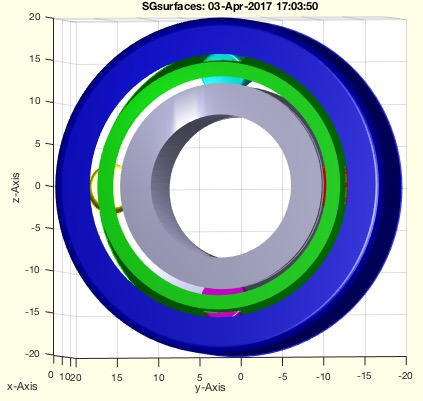% SGsurfaces(SG,slct) - returns a cell list of separated CLOSED surfaces similar to SGseparate% (by Tim Lueth, VLFL-Lib, 2017-APR-03 as class: SURFACES)% % SGsurfaces and SGseparate analyse independent closed surfaces.% SGanalyzeGroupParts analyses penetration of surfaces.% For open surfaces use surfacesofSG (Status of: 2017-07-07)% % Introduced first in SolidGeometry 3.8% % See also: SGsurfaceplot, SGsurfacehistogram, SGseparate, SGpacking, % SGwriteSeparatedSTL, SGanalyzeGroupParts, surfacesofSG% % [SGC,n]=SGsurfaces(SG,[slct])% === INPUT PARAMETERS ===% SG: Solid geoemtry as single struct% slct: surface index; default is all; SGseparate shows all% === OUTPUT RESULTS ======% SGC: cell list of solid geometry structs% n: length of cell list% % EXAMPLE: % SGsurfaces(SGsample(17))% SGwriteSeparatedSTL(SGsurfaces(SGsample(17)))% SGpacking(SGsurfaces(SGsample(17)))% % See also: SGsurfaceplot, SGsurfacehistogram, SGseparate, SGpacking, % SGwriteSeparatedSTL, SGanalyzeGroupParts, surfacesofSG%
###### VMintensityscale(V,cax)- returns an intensity scaled voxel image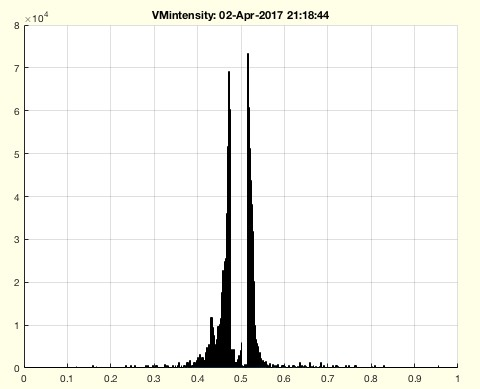% VMintensityscale(V,cax) - returns an intensity scaled voxel image% (by Tim Lueth, VLFL-Lib, 2017-APR-02 as class: VOXELS)% % See also: VMplot, VMmontage, VMresize% % [c,V]=VMintensityscale(V,[cax])% === INPUT PARAMETERS ===% V: Voxel mode% cax: desired intensity interval; default is [0 1]% === OUTPUT RESULTS ======% c: [minc maxc]% V: scaled to 0 to 1%
###### getprojectionimage(pc,vdist,vwidth,cupv)- returns a single projection image of gca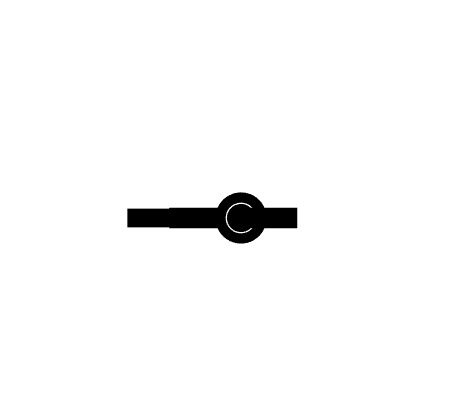% getprojectionimage(pc,vdist,vwidth,cupv) - returns a single projection image of gca% (by Tim Lueth, VLFL-Lib, 2017-APR-02 as class: ANALYTICAL GEOMETRY)% % See also: getgcapixelsize, projectionimage% % I=getprojectionimage(pc,vdist,vwidth,cupv)% === INPUT PARAMETERS ===% pc: camera position [-x y z] with direction through [0 0 0]% vdist: distance of the screen behind [0 0 0]% vwidth: length of the screen in y and z% cupv: camera up vector; default is [0 -1 0]% === OUTPUT RESULTS ======% I: Image calculated by getframe and getgcapixelsize%
###### getgcapixelsize- returns the position of the gca in the current figure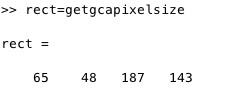% getgcapixelsize - returns the position of the gca in the current figure% (by Tim Lueth, VLFL-Lib, 2017-APR-02 as class: USER INTERFACE)% % This fnctn is required if getframe(gca) does not work anymore. % In this case try getframe(gcf,getgcapixelsize) (Status of: 2017-04-02)% % See also: setgcapixelsize% % rect=getgcapixelsize% === OUTPUT RESULTS ======% rect: [left bottom width height] in pixels% % EXAMPLE: % subplot(2,2,2)% rect=getgcapixelsize% I=getframe(gcf,rect); figure(3333); imshow(I.cdata);%
###### projectionimage(pc,vdist,vwidth,pixel,w)- returns a central projection image by using Matlabs view commands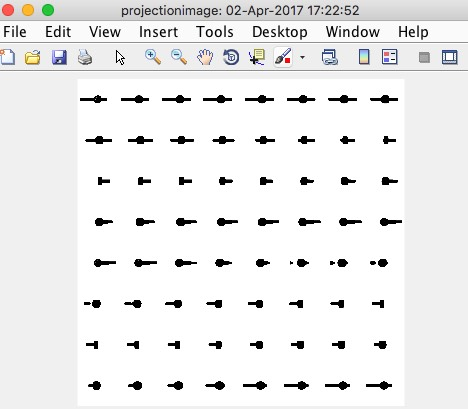% projectionimage(pc,vdist,vwidth,pixel,w) - returns a central projection image by using Matlabs view commands% (by Tim Lueth, Video-Lib, 2017-APR-02 as class: ANALYTICAL GEOMETRY)% % this fnctn returns a projection image of the current gca. The % projections is always done in relation to the point [0 0 0]; The target % is always% % It changes a lot of view parameter such as lighting shading etc.% It can be used to create virtual conebeam images (Status of: 2017-04-02)% % See also: setgcapixelsize, VLprojection% % [I,cax,pc]=projectionimage(pc,vdist,vwidth,[pixel,w])% === INPUT PARAMETERS ===% pc: camera view point% vdist: camera target point% vwidth: virtual screen width% pixel: pixel size of virtual screen% w: single angle value or list an angles; default is 0% === OUTPUT RESULTS ======% I: Image of pixel size or image stack% cax: color axis [min max]% pc: center point or list of center points% % EXAMPLE: % SGfigure; SGsample(17); view(-30,30);% projectionimage([0 0 -10],10,200,512,0:pi/32:2*pi-1e-3); % central % beam projection% [I,ca]=projectionimage([0 0 -10],10,200,512,0:pi/32:2*pi-1e-3);% projectionimage([0 0 -100000],10,200,512); % parallel projection%
###### setgcapixelsize(siz)- sets the current gca to a default pixelsize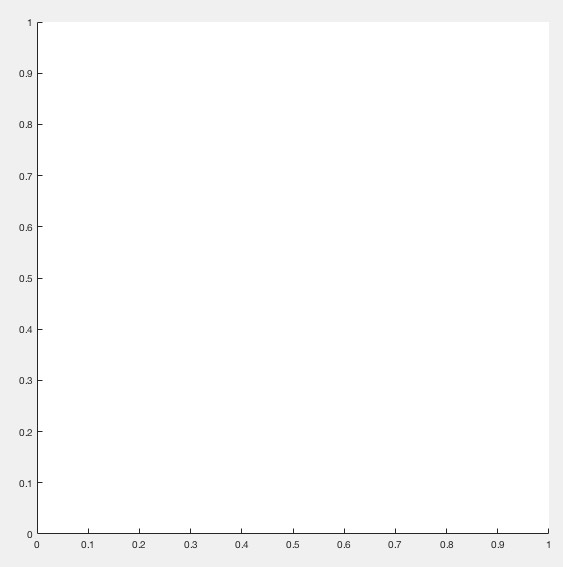% setgcapixelsize(siz) - sets the current gca to a default pixelsize% (by Tim Lueth, VLFL-Lib, 2017-APR-01 as class: ANALYTICAL GEOMETRY)% % This fnctn is helpful if projections should be calculated on pixel % level as used in conebeam or ct projections (Status of: 2017-04-01)% % See also: getgcapixelsize% % ns=setgcapixelsize(siz)% === INPUT PARAMETERS ===% siz: [ x y], i.e. [c r]% === OUTPUT RESULTS ======% ns: [x y], i.e. [c r]% % EXAMPLE: % setgcapixelsize([512 512])%
###### VLprojection(SG,VL,ez,cent)- returns projected vertex lists on a surface/solid for given vertex list% VLprojection(SG,VL,ez,cent) - returns projected vertex lists on a surface/solid for given vertex list % (by Tim Lueth, VLFL-Lib, 2017-APR-01 as class: EXPERIMENTS)% % The projection surface is given as a solid. Now either by parallel % projection or central projection the resulting vertex posions on the % surface are returned. Also the distance and the index of the hit % triangles are returned. It is important to understand that the use of % EL or FL needs an additional handling, since in this case additional % points have to be created similar to SGbool.% There is also another possibility to calculate projections on % pixel/voxel accuracy using view and axes. (Status of: 2017-04-01)% % Introduced first in SolidGeometry 3.8% % See also: VLFLpprojectPL, VLFLpprojectPLEL, crosspointVLFL, % intersectstriangle% % [VLN,DL,fi]=VLprojection([SG,VL,ez,cent])% === INPUT PARAMETERS ===% SG: SG.VL, SG.FL; solid or surface as projection plane% VL: CPL,CVL or VL as vertex list to project% ez: beam direction (cent=false) or beam origin (cent=true)% cent: central (true) or parallel projection (false); default = false;% === OUTPUT RESULTS ======% VLN: Projected vertex list% DL: Distance list for each vertex% fi: facet index list for each point% % EXAMPLE: Try central and parallel projection% SG=SGtransP(SGbox([80,80,5]),[0 0 40]); VL=VLaddz(CPLsample(12));% VLprojection(SG,VL,[0 0 1],false) % parallel projection% VLprojection(SG,VL,[0 0 -30],true) % central projection% % See also: VLFLpprojectPL, VLFLpprojectPLEL, crosspointVLFL, % intersectstriangle%
###### exp_2017_03_30(A)- show the bounding box faces that are touched by the solid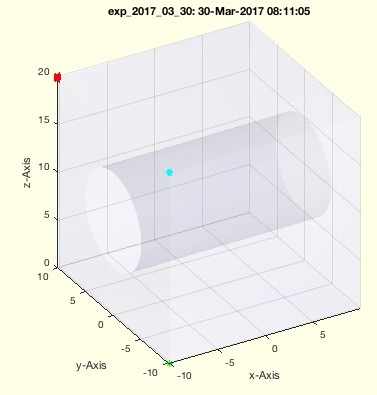% exp_2017_03_30(A) - show the bounding box faces that are touched by the solid% (by Tim Lueth, VLFL-Lib, 2017-MÄR-30 as class: EXPERIMENTS)% % If STL files have been created using CATIA sometimes the Solids are % rotated slightly. This can be corrected by detecting plane surfaces and % adjust them by inverse transformation of the plane coordinate system. % Nevertheless, it is difficult to see% This fnctn is an example how to visualize the problem (Status of: % 2017-03-30)% % [XL,YL,ZL]=exp_2017_03_30(A)% === INPUT PARAMETERS ===% A: Solid% === OUTPUT RESULTS ======% XL: List of coordinate that define the X-plane% YL: List of coordinate that define the Y-plane% ZL: List of coordinate that define the Z-plane%